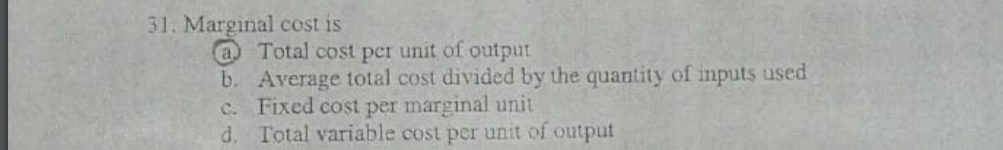# 31. Marginal cost is a Total cost per unit of output b. Average total cost divided by the quantity of inputs used c. Fixed cost per marginal unit d. Total variable cost per unit of output

Questionhelp_outlineImage Transcriptionclose31. Marginal cost is a Total cost per unit of output b. Average total cost divided by the quantity of inputs used c. Fixed cost per marginal unit d. Total variable cost per unit of output fullscreen

### Want to see this answer and more?

Experts are waiting 24/7 to provide step-by-step solutions in as fast as 30 minutes!*

*Response times may vary by subject and question complexity. Median response time is 34 minutes for paid subscribers and may be longer for promotional offers.
Tagged in